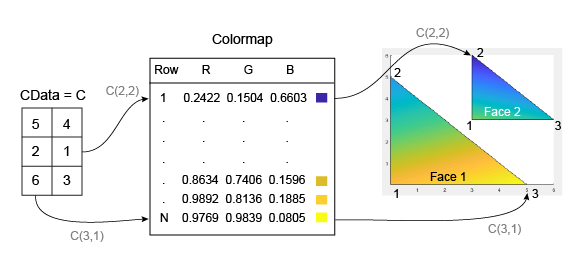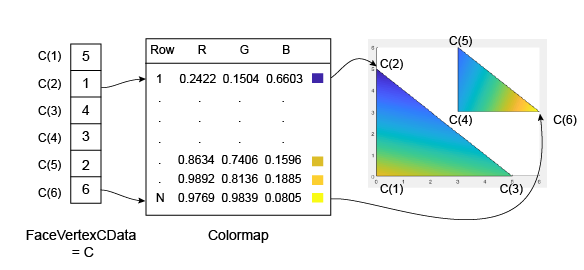## How Patch Data Relates to a Colormap

When you create graphics that use `Patch` objects, you can control the overall color scheme by calling the `colormap` function. You can also control the relationship between the colormap and your patch by:

• Assigning specific colors to the faces

• Assigning specific colors to the vertices surrounding each face

The way you control these relationships depends on how you specify your patches: as x-, y-, and z-coordinates, or as face-vertex data.

### Relationship of the Colormap to x-, y-, and z-Coordinate Arrays

If you create a `Patch` object using x-, y-, and z-coordinate arrays, the `CData` property of the `Patch` object contains an indexing array `C`. This array controls the relationship between the colormap and your patch. To assign colors to the faces, specify `C` as an array with these characteristics:

• `C` is an n-by-1 array, where n is the number of faces.

• The value at `C(i)` controls the color for face `i`.

Here is an example of `C` and its relationship to the colormap and three faces. The value of `C(i)` controls the color for the face defined by vertices (`X(i,:)`, `Y(i,:)`).The smallest value in `C` is `0`. It maps to the first row in the colormap. The largest value in `C` is `1`, and it maps to the last row in the colormap. Intermediate values of `C` map linearly to the intermediate rows in the colormap. In this case, `C(2)` maps to the color located about two-thirds from the beginning of the colormap. This code creates the `Patch` object described in the preceding illustration.

```X = [0 0 5; 0 0 5; 4 4 9]; Y = [0 4 0; 3 7 3; 0 4 0]; C = [0; .6667; 1]; p = patch(X,Y,C); colorbar```To assign colors to the vertices, specify `C` as an array with these characteristics:

• `C` is an m-by-n array, where m is the number of vertices per face, and n is the number of faces.

• The value at `C(i,j)` controls the color at vertex `i` of face `j`.

Here is an example of `C` and its relationship to the colormap and six vertices. The value of `C(i,j)` controls the color for the vertex at (`X(i,j)`, `Y(i,j)`).As with patch faces, MATLAB® scales the values in `C` to the number of rows in the colormap. In this case, the smallest value is `C(2,2)=1`, and it maps to the first row in the colormap. The largest value is `C(3,1)=6`, and it maps to the last row in the colormap.

This code creates the `Patch` object described in the preceding illustration. The `FaceColor` property is set to `'interp'` to make the vertex colors blend across each face.

```clf X = [0 3; 0 3; 5 6]; Y = [0 3; 5 6; 0 3]; C = [5 4; 2 0; 6 3]; p = patch(X,Y,C,'FaceColor','interp'); colorbar```### Relationship of the Colormap to Face-Vertex Data

If you create patches using face-vertex data, the `FaceVertexCData` property of the `Patch` object contains an indexing array `C`. This array controls the relationship between the colormap and your patch.

To assign colors to the faces, specify `C` as an array with these characteristics:

• `C` is an n-by-1 array, where n is the number of faces.

• The value at `C(i)` controls the color for face `i`.

Here is an example of `C` and its relationship to the colormap and three faces.The smallest value in `C` is `0`, and it maps to the first row in the colormap. The largest value in `C` is `1`, and it maps to the last value in the colormap. Intermediate values of `C` map linearly to the intermediate rows in the colormap. In this case, `C(2)` maps to the color located about two-thirds from the bottom of the colormap.

This code creates the `Patch` object described in the preceding illustration. The `FaceColor` property is set to `'flat'` to display the colormap colors instead of the default color, which is black.

```clf vertices = [0 0; 0 3; 4 0; 0 4; 0 7; 4 4; 5 0; 5 3; 9 0]; faces = [1 2 3; 4 5 6; 7 8 9]; C = [0; 0.6667; 1]; p = patch('Faces',faces,'Vertices',vertices,'FaceVertexCData',C); p.FaceColor = 'flat'; colorbar```To assign colors to the vertices, specify the `FaceVertexCData` property of the `Patch` object as array `C` with these characteristics:

• `C` is an n-by-1 array, where n is the number of vertices.

• The value at `C(i)` controls the color at vertex `i`.

Here is an example of `C` and its relationship to the colormap and six vertices.As with patch faces, MATLAB scales the values in `C` to the number of rows in the colormap. In this case, the smallest value is `C(2)=1`, and it maps to the first row in the colormap. The largest value is `C(6)=6`, and it maps to the last row in the colormap.

This code creates the `Patch` object described in the preceding illustration. The `FaceColor` property is set to `'interp'` to make the vertex colors blend across each face.

```clf vertices = [0 0; 0 5; 5 0; 3 3; 3 6; 6 3]; faces = [1 2 3; 4 5 6]; C = [5; 1; 4; 3; 2; 6]; p = patch('Faces',faces,'Vertices',vertices,'FaceVertexCData',C); p.FaceColor = 'interp'; colorbar```